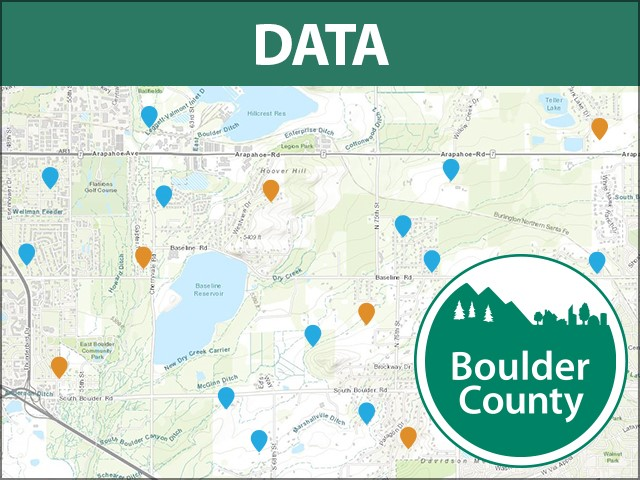Description

more
Following the 2013 flood, the Colorado Water Conservation Board (CWCB) initiated a program to re-map the predicted 1% chance regulatory floodplain (100-year flood zone) of the most affected waterways. The program was named the "Colorado Hazard Mapping Program" or "CHAMP." This has been adopted by the Boulder County Commissioners, and will be used for regulatory decisions by the county. Not necessarily used for determining flood insurance.

Dataset Attributes

• OBJECTID
Number
• DFIRM_ID
Text
{"value"=>"08013C", "count"=>3298} (), {"value"=>"08059C", "count"=>306} (), {"value"=>"08123C", "count"=>288} (), {"value"=>"08047C", "count"=>190} (), {"value"=>"08069C", "count"=>76} (), {"value"=>"08031C", "count"=>4} ()
• VERSION_ID
Text
{"value"=>"2.3.3.4", "count"=>4162} ()
• FLD_AR_ID
Text
{"value"=>"4004", "count"=>629} (), {"value"=>"16618", "count"=>533} (), {"value"=>"15388", "count"=>355} (), {"value"=>"2293", "count"=>98} (), {"value"=>"1634", "count"=>60} (), {"value"=>"0", "count"=>46} (), {"value"=>"1632", "count"=>38} (), {"value"=>"11295", "count"=>23} (), {"value"=>"774", "count"=>14} (), {"value"=>"16336", "count"=>14} ()
• STUDY_TYP
Text
{"value"=>"SFHAs WITH HIGH FLOOD RISK", "count"=>1923, "code"=>"1020"} (), {"value"=>"SFHAs WITH LOW FLOOD RISK", "count"=>772, "code"=>"1000"} (), {"value"=>"SFHAs WITH LOW FLOOD RISK", "count"=>712, "code"=>"SFHAs WITH LOW FLOOD RISK"} (), {"value"=>"SFHAs WITH MEDIUM FLOOD RISK", "count"=>547, "code"=>"SFHAs WITH MEDIUM FLOOD RISK"} (), {"value"=>"SFHAs WITH HIGH FLOOD RISK", "count"=>195, "code"=>"SFHAs WITH HIGH FLOOD RISK"} (), {"value"=>"SFHAs WITH MEDIUM FLOOD RISK", "count"=>17, "code"=>"1010"} ()
• FLD_ZONE
Text
{"value"=>"AE", "count"=>2395, "code"=>"AE"} (), {"value"=>"X", "count"=>1786, "code"=>"X"} (), {"value"=>"A", "count"=>27, "code"=>"A"} (), {"value"=>"AO", "count"=>7, "code"=>"AO"} ()
• ZONE_SUBTY
Text
{"value"=>"0.2 PCT ANNUAL CHANCE FLOOD HAZARD", "count"=>1679, "code"=>"0500"} (), {"value"=>"FLOODWAY", "count"=>1458, "code"=>"1100"} (), {"value"=>"AREA OF MINIMAL FLOOD HAZARD", "count"=>67, "code"=>"2000"} (), {"value"=>"AREA WITH REDUCED FLOOD RISK DUE TO LEVEE", "count"=>40, "code"=>"1000"} ()
• SFHA_TF
Text
{"value"=>"T", "count"=>2377, "code"=>"T"} (), {"value"=>"F", "count"=>1786, "code"=>"F"} ()
• STATIC_BFE
Number
-9999 to 0
• V_DATUM
Text
{"value"=>" ", "count"=>2903, "code"=>" "} (), {"value"=>"NAVD88", "count"=>1007, "code"=>"NAVD88"} ()
• DEPTH
Number
-9999 to 1
• LEN_UNIT
Text
{"value"=>" ", "count"=>3734, "code"=>" "} (), {"value"=>"<Null>", "count"=>169, "code"=>"<Null>"} (), {"value"=>"Feet", "count"=>2, "code"=>"FT"} ()
• VELOCITY
Number
-9999 to 0
• VEL_UNIT
Text
{"value"=>" ", "count"=>3734, "code"=>" "} (), {"value"=>"<Null>", "count"=>169, "code"=>"<Null>"} ()
• AR_REVERT
Text
{"value"=>" ", "count"=>3734, "code"=>" "} (), {"value"=>"<Null>", "count"=>169, "code"=>"<Null>"} ()
• AR_SUBTRV
Text
{"value"=>" ", "count"=>3688, "code"=>" "} (), {"value"=>"<Null>", "count"=>169, "code"=>"<Null>"} ()
• BFE_REVERT
Number
-9999 to 0
• DEP_REVERT
Number
-9999 to 0
• DUAL_ZONE
Text
{"value"=>" ", "count"=>3688, "code"=>" "} ()
• SOURCE_CIT
Text
{"value"=>"STUDY1", "count"=>3060} (), {"value"=>"STUDY2", "count"=>187} (), {"value"=>"Tie in", "count"=>2} (), {"value"=>"Tie-in", "count"=>1} ()
• REGULATING_ENTITY
Text
{"value"=>"Boulder County", "count"=>4215} ()
• SHAPE
Number
• SHAPE.STArea()
Number
• SHAPE.STLength()
Number

• By on June 1, 2017
• Updated 3 months ago

Related Datasets

• Floodplain - FEMA RegulatedFloodplain - FEMA Regulated
• Mobile HomesMobile Homes
• Road Closures TEST• Fourmile Fire Perimeter - September 13th 2010